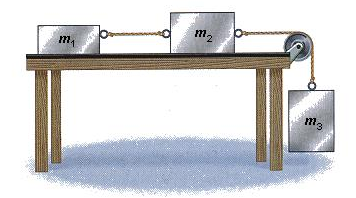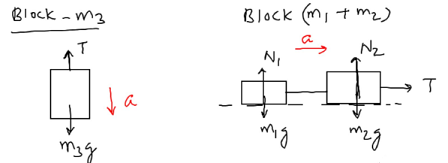# Find the acceleration of the masses shown in the following figure, given that m1 = 0.65 kg, m2 =...

## Question:

. Find the acceleration of the masses shown in the following figure, given that {eq}m_1 = 0.65\ kg {/eq} , {eq}m_2 = 1.40\ kg {/eq}, and {eq}m_3 = 2.55\ kg {/eq}. (Assume the table is frictionless).## Newton's Second Law of Motion:

When an object is under the action of various external forces, the net resultant force of all the forces produces acceleration of the object. According to Newton's Second Law, the expression is written as:

• {eq}\rm Net\ external\ force = mass\times acceleration{/eq}

## Answer and Explanation:

We are given:

Three blocks of masses as shown in the given figure:
{eq}*m_1 = 0.65\ kg\\*m_2 = 1.40\ kg\\*m_3 = 2.55\ kg{/eq}

The free body diagram is shown below:Finding the acceleration of the block

Referring to the free body diagram above, and applying Newton's Second Law of Motion:

Block {eq}m_3{/eq}:

• {eq}m_3\ g - T = m_3\ a{/eq} ----Equation - 1

Block {eq}(m_1 + m_2){/eq}:

• {eq}T = (m_1 + m_2)\ a{/eq} ----Equation - 2

From Equations - 1 and 2

• {eq}a = \dfrac{m_3\ g}{(m_1 + m_2 + m_3)}{/eq}
• {eq}a = \dfrac{2.55\times 9.8}{(0.65 + 1.40 + 2.55)}{/eq}
• {eq}a = 5.43\ m/s^2{/eq}

#### Learn more about this topic:Practice Applying Newton's Second Law

from Physics 111: Physics I

Chapter 6 / Lesson 9
2.5K